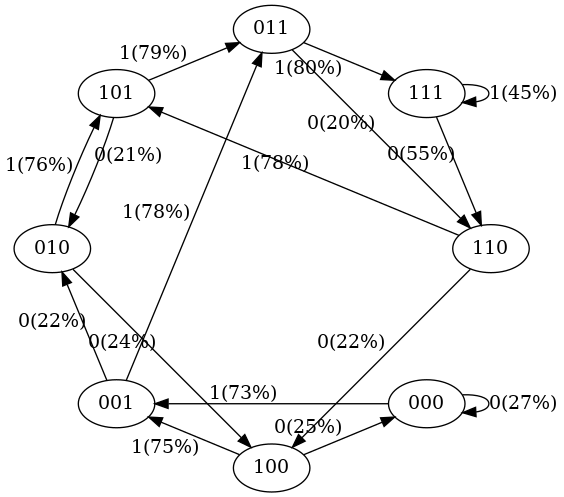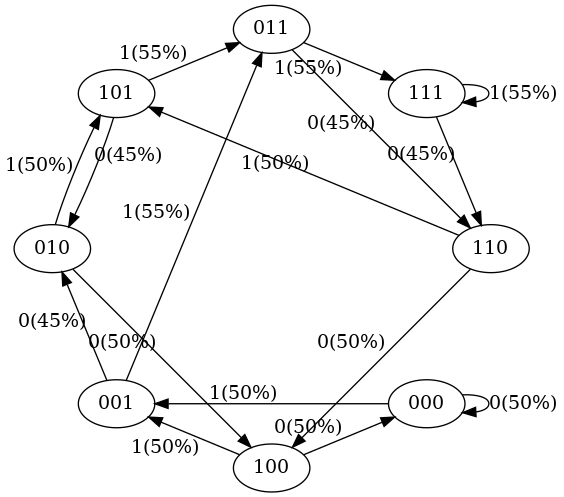# [译] GPT 是如何工作的：200 行 Python 代码实现一个极简 GPT（2023）

Published at 2023-05-21 | Last Update 2023-08-12

### 译者序

Andrej Karpathy 博士 2015 毕业于斯坦福，之后先在 OpenAI 待了两年，是 OpenAI 的创始成员和研究科学家，2017 年加入 Tesla，带领 Tesla Autopilot 团队， 2022 年离职后在 Youtube 上科普人工智能相关技术，2023 年重新回归 OpenAI。

# 摘要1. 在训练数据中，状态 `101 -> 011` 的概率是 100%，因此我们看到训练之后的模型中， `101 -> 011 `的转移概率很高（79%，没有达到 100% 是因为我们只做了 50 步迭代）；
2. 在训练数据中，状态 `111 -> 111``111 -> 110` 的概率分别是 50%； 在训练之后的模型中，两个转移概率分别为 45% 和 55%，也差不多是一半一半；
3. 在训练数据中没有出现 `000` 这样的状态，在训练之后的模型中， 它转移到 `001``000` 的概率并不是平均的，而是差异很大（73% 到 001，27% 到 000）， 这是 Transformer 内部 inductive bias 的结果，也符合预期。

# 1 引言

GPT 是一个神经网络，根据输入的 token sequence（例如，`1234567`） 来预测下一个 token 出现的概率

## 1.1 极简 GPT：token 只有 0 和 1

• 输入：由 0 和 1 组成的一串 token，例如 `100011111`
• 输出：“下一个 token 是 0 的概率”（`P(0)`）和“下一个 token 是 1 的概率”（`P(1)`）。

• P(0) = 20%
• P(1) = 80%

## 1.2 状态（上下文）和上下文长度

• 三个 token 就组成这个 GPT 的一个上下文（context），也是 GPT 的一个状态
• `3` 就是上下文长度（context length）

## 1.3 状态空间

• `vocab_size`（vocabulary size，字典空间）：单个 token 有多少种可能的值， 例如上面提到的 binary GPT 每个 token 只有 0 和 1 这两个可能的取值；
• `context_length`：上下文长度，用 `token` 个数来表示，例如 3 个 token。

### 1.3.1 简化版状态空间

`total_states = vocab_sizecontext_length`

• `vocab_size = 2`：token 可能的取值是 `0``1`，总共两个；
• `context_length = 3 tokens`：上下文长度是 3 个 token；

### 1.3.2 真实版状态空间

• token sequence 长度为 1：总共 2^1 = 2 个状态
• token sequence 长度为 2：总共 2^2 = 4 个状态
• token sequence 长度为 3：总共 2^3 = 8 个状态

## 1.4 状态转移

• 正面朝上表示 token=1，反面朝上表示 token=0；
• 新来一个 token 时，将更新 context：将新 token 追加到最右边，然后把最左边的 token 去掉，从而得到一个新 context；

## 1.5 马尔科夫链

• Token sequence（例如 [0,1,0]）组成状态集合，
• 从一个状态到另一个状态的转换是转移概率。

# 2 准备工作

## 2.1 安装 pytorch

``````\$ pip3 install torch torchvision -i https://pypi.mirrors.ustc.edu.cn/simple # 用国内源加速
\$ pip3 install graphviz -i https://pypi.mirrors.ustc.edu.cn/simple
``````

## 2.2 BabyGPT 源码 `babygpt.py`

``````#@title minimal GPT implementation in PyTorch
""" super minimal decoder-only gpt """

import math
from dataclasses import dataclass
import torch
import torch.nn as nn
from torch.nn import functional as F

torch.manual_seed(1337)

class CausalSelfAttention(nn.Module):
def __init__(self, config):
super().__init__()
assert config.n_embd % config.n_head == 0
# key, query, value projections for all heads, but in a batch
self.c_attn = nn.Linear(config.n_embd, 3 * config.n_embd, bias=config.bias)
# output projection
self.c_proj = nn.Linear(config.n_embd, config.n_embd, bias=config.bias)
# regularization
self.n_embd = config.n_embd
self.register_buffer("bias", torch.tril(torch.ones(config.block_size, config.block_size))
.view(1, 1, config.block_size, config.block_size))

def forward(self, x):
B, T, C = x.size() # batch size, sequence length, embedding dimensionality (n_embd)

# calculate query, key, values for all heads in batch and move head forward to be the batch dim
q, k ,v  = self.c_attn(x).split(self.n_embd, dim=2)
k = k.view(B, T, self.n_head, C // self.n_head).transpose(1, 2) # (B, nh, T, hs)
q = q.view(B, T, self.n_head, C // self.n_head).transpose(1, 2) # (B, nh, T, hs)
v = v.view(B, T, self.n_head, C // self.n_head).transpose(1, 2) # (B, nh, T, hs)

# manual implementation of attention
att = (q @ k.transpose(-2, -1)) * (1.0 / math.sqrt(k.size(-1)))
att = att.masked_fill(self.bias[:,:,:T,:T] == 0, float('-inf'))
att = F.softmax(att, dim=-1)
y = att @ v # (B, nh, T, T) x (B, nh, T, hs) -> (B, nh, T, hs)
y = y.transpose(1, 2).contiguous().view(B, T, C) # re-assemble all head outputs side by side

# output projection
y = self.c_proj(y)
return y

class MLP(nn.Module):
def __init__(self, config):
super().__init__()
self.c_fc    = nn.Linear(config.n_embd, 4 * config.n_embd, bias=config.bias)
self.c_proj  = nn.Linear(4 * config.n_embd, config.n_embd, bias=config.bias)
self.nonlin = nn.GELU()

def forward(self, x):
x = self.c_fc(x)
x = self.nonlin(x)
x = self.c_proj(x)
return x

class Block(nn.Module):
def __init__(self, config):
super().__init__()
self.ln_1 = nn.LayerNorm(config.n_embd)
self.attn = CausalSelfAttention(config)
self.ln_2 = nn.LayerNorm(config.n_embd)
self.mlp = MLP(config)

def forward(self, x):
x = x + self.attn(self.ln_1(x))
x = x + self.mlp(self.ln_2(x))
return x

@dataclass
class GPTConfig:
# 以下是 GPT-2 的 hyperparameters，后面我们的 BabyGPT 初始化时，会用更小的值覆盖掉默认值
block_size: int = 1024
vocab_size: int = 50304 # 字典：5 万多个唯一的 token，包括单词（3000 多个常用单词）、词根、标点等等
n_layer: int = 12       # 12 层神经网络
n_embd: int = 768       # 嵌入向量（特征向量）的维度：每个 token 都用一个 768x1 数组表示
bias: bool = False

class GPT(nn.Module):
def __init__(self, config):
super().__init__()
assert config.vocab_size is not None
assert config.block_size is not None
self.config = config

self.transformer = nn.ModuleDict(dict(
wte = nn.Embedding(config.vocab_size, config.n_embd),
wpe = nn.Embedding(config.block_size, config.n_embd),
h = nn.ModuleList([Block(config) for _ in range(config.n_layer)]),
ln_f = nn.LayerNorm(config.n_embd),
))

# init all weights
self.apply(self._init_weights)
# apply special scaled init to the residual projections, per GPT-2 paper
for pn, p in self.named_parameters():
if pn.endswith('c_proj.weight'):
torch.nn.init.normal_(p, mean=0.0, std=0.02/math.sqrt(2 * config.n_layer))

# report number of parameters
print("number of parameters: %d" % (sum(p.nelement() for p in self.parameters()),))

def _init_weights(self, module):
if isinstance(module, nn.Linear):
torch.nn.init.normal_(module.weight, mean=0.0, std=0.02)
if module.bias is not None:
torch.nn.init.zeros_(module.bias)
elif isinstance(module, nn.Embedding):
torch.nn.init.normal_(module.weight, mean=0.0, std=0.02)

def forward(self, idx):
device = idx.device
b, t = idx.size()
assert t <= self.config.block_size, f"Cannot forward sequence of length {t}, block size is only {self.config.block_size}"
pos = torch.arange(0, t, dtype=torch.long, device=device).unsqueeze(0) # shape (1, t)

# forward the GPT model itself
tok_emb = self.transformer.wte(idx) # token embeddings of shape (b, t, n_embd)
pos_emb = self.transformer.wpe(pos) # position embeddings of shape (1, t, n_embd)
x = tok_emb + pos_emb
for block in self.transformer.h:
x = block(x)
x = self.transformer.ln_f(x)
logits = self.lm_head(x[:, -1, :]) # note: only returning logits at the last time step (-1), output is 2D (b, vocab_size)
return logits
``````

# 3 基于 BabyGPT 创建一个 binary GPT

## 3.1 设置 GPT 参数

``````# hyperparameters for our GPT
vocab_size = 2     # 词汇表 size 为 2，因为我们只有两个可能的 token：0 和 1
context_length = 3 # 上下文长度位 3，即只用 3 个 bit 来预测下一个 token 出现的概率

config = GPTConfig(
block_size = context_length,
vocab_size = vocab_size,
n_layer = 4, # 这个以及接下来几个参数都是 Transformer 神经网络的 hyperparameters，
n_head = 4,  # 不理解没关系，认为是 GPT 的默认参数就行了。
n_embd = 16,
bias = False,
)
``````

## 3.2 随机初始化

``````gpt = GPT(config)
``````

``````Number of parameters: 12656
``````

### 3.2.1 查看初始状态和转移概率

``````def possible_states(n, k):
# return all possible lists of k elements, each in range of [0,n)
if k == 0:
yield []
else:
for i in range(n):
for c in possible_states(n, k - 1):
yield [i] + c

list(possible_states(vocab_size, context_length))
``````

``````def plot_model():
dot = Digraph(comment='Baby GPT', engine='circo')

print("\nDump BabyGPT state ...")
for xi in possible_states(gpt.config.vocab_size, gpt.config.block_size):
# forward the GPT and get probabilities for next token
x = torch.tensor(xi, dtype=torch.long)[None, ...] # turn the list into a torch tensor and add a batch dimension
# tensor 是一个多维矩阵数据结构

logits = gpt(x) # forward the gpt neural net
probs = nn.functional.softmax(logits, dim=-1) # get the probabilities
y = probs.tolist() # remove the batch dimension and unpack the tensor into simple list
print(f"input {xi} ---> {y}")

# also build up the transition graph for plotting later
current_node_signature = "".join(str(d) for d in xi)
dot.node(current_node_signature)
for t in range(gpt.config.vocab_size):
next_node = xi[1:] + [t] # crop the context and append the next character
next_node_signature = "".join(str(d) for d in next_node)
p = y[t]
label=f"{t}({p*100:.0f}%)"
dot.edge(current_node_signature, next_node_signature, label=label)
return dot
``````

``````#      输入状态      输出概率 [P(0),      P(1)              ]
input [0, 0, 0] ---> [0.4963349997997284, 0.5036649107933044]
input [0, 0, 1] ---> [0.4515703618526459, 0.5484296679496765]
input [0, 1, 0] ---> [0.49648362398147583, 0.5035163760185242]
input [0, 1, 1] ---> [0.45181113481521606, 0.5481888651847839]
input [1, 0, 0] ---> [0.4961162209510803, 0.5038837194442749]
input [1, 0, 1] ---> [0.4517717957496643, 0.5482282042503357]
input [1, 1, 0] ---> [0.4962802827358246, 0.5037197470664978]
input [1, 1, 1] ---> [0.4520467519760132, 0.5479532480239868]
``````

### 3.2.2 状态转移图• 在每个状态下，下一个 token 只有 0 和 1 两种可能，因此每个节点有 2 个出向箭头；
• 每个状态的入向箭头数量不完全一样；
• 每次状态转换时，最左边的 token 被丢弃，新 token 会追加到最右侧，这个前面也介绍过了；
• 另外注意到，此时的状态转移概率大部分都是均匀分布的（这个例子中是 50%）， 这也符合预期，因为我们还没拿真正的输入序列（不是初始的 8 个状态）来训练这个模型

## 3.3 训练

### 3.3.1 输入序列预处理

``````Python 3.8.2 (default, Mar 13 2020, 10:14:16)
>>> seq = list(map(int, "111101111011110"))
>>> seq
[1, 1, 1, 1, 0, 1, 1, 1, 1, 0, 1, 1, 1, 1, 0]
``````

``````def get_tensor_from_token_sequence():
X, Y = [], []

# iterate over the sequence and grab every consecutive 3 bits
# the correct label for what's next is the next bit at each position
for i in range(len(seq) - context_length):
X.append(seq[i:i+context_length])
Y.append(seq[i+context_length])
print(f"example {i+1:2d}: {X[-1]} --> {Y[-1]}")
X = torch.tensor(X, dtype=torch.long)
Y = torch.tensor(Y, dtype=torch.long)
print(X.shape, Y.shape)

get_tensor_from_token_sequence()
``````

``````example  1: [1, 1, 1] --> 1
example  2: [1, 1, 1] --> 0
example  3: [1, 1, 0] --> 1
example  4: [1, 0, 1] --> 1
example  5: [0, 1, 1] --> 1
example  6: [1, 1, 1] --> 1
example  7: [1, 1, 1] --> 0
example  8: [1, 1, 0] --> 1
example  9: [1, 0, 1] --> 1
example 10: [0, 1, 1] --> 1
example 11: [1, 1, 1] --> 1
example 12: [1, 1, 1] --> 0
torch.Size([12, 3]) torch.Size()
``````

### 3.3.2 开始训练

``````def do_training(X, Y):
# init a GPT and the optimizer
torch.manual_seed(1337)
gpt = babygpt.GPT(config)

# train the GPT for some number of iterations
for i in range(50):
logits = gpt(X)
loss = F.cross_entropy(logits, Y)
loss.backward()
optimizer.step()
print(i, loss.item())

do_training(X, Y)
``````

``````0 0.663539469242096
1 0.6393510103225708
2 0.6280076503753662
3 0.6231870055198669
4 0.6198631525039673
5 0.6163331270217896
6 0.6124278903007507
7 0.6083487868309021
8 0.6043017506599426
9 0.6004215478897095
10 0.5967749953269958
11 0.5933789610862732
12 0.5902208685874939
13 0.5872761011123657
14 0.5845204591751099
15 0.5819371342658997
16 0.5795179009437561
17 0.5772626996040344
18 0.5751749873161316
19 0.5732589960098267
20 0.5715171694755554
21 0.5699482560157776
22 0.5685476660728455
23 0.5673080086708069
24 0.5662192106246948
25 0.5652689337730408
26 0.5644428730010986
27 0.563723087310791
28 0.5630872845649719
29 0.5625078678131104
30 0.5619534254074097
31 0.5613844990730286
32 0.5607481598854065
33 0.5599767565727234
34 0.5589826107025146
35 0.5576505064964294
36 0.5558211803436279
37 0.5532580018043518
38 0.5495675802230835
39 0.5440602898597717
40 0.5359978079795837
41 0.5282725095748901
42 0.5195847153663635
43 0.5095029473304749
44 0.5019271969795227
45 0.49031805992126465
46 0.48338067531585693
47 0.4769590198993683
48 0.47185763716697693
49 0.4699831008911133
``````

### 3.3.3 训练之后的状态转移概率图• 101 总是转换为 011：经过 50 次训练之后，我们看到这种转换有 `79%` 的概率；
• 111 在 50% 的时间内变为 111，在 50% 的时间内变为 110：训练之后概率分别是 45% 和 55%。

1. 没有看到 100% 或 50% 的转移概率：

这是因为神经网络没有经过充分训练，继续训练就会出现更接近这两个值的转移概率；

2. 训练数据中没出现过的状态（例如 000 或 100），转移到下一个状态的概率 （预测下一个 token 是 0 还是 1 的概率）并不是均匀的`50% vs. 50%`）， 而是差异很大（上图中是 `75% vs. 25%`）。

如果训练期间从未遇到过这些状态，那它们的转移概率不应该在 ~50% 吗？ 不是，以上结果也是符合预期的。因为在真实部署场景中，GPT 的几乎每个输入都没有在训练中见过。 这种情况下，我们依靠 GPT 自身内部设计及其 inductive bias 来执行适当的泛化。

## 3.4 采样（推理）

``````xi = [1, 1, 1] # the starting sequence
fullseq = xi.copy()
print(f"init: {xi}")
for k in range(20):
x = torch.tensor(xi, dtype=torch.long)[None, ...]
logits = gpt(x)
probs = nn.functional.softmax(logits, dim=-1)
t = torch.multinomial(probs, num_samples=1).item() # sample from the probability distribution
xi = xi[1:] + [t] # transition to the next state
fullseq.append(t)
print(f"step {k}: state {xi}")

print("\nfull sampled sequence:")
print("".join(map(str, fullseq)))
``````

``````init: [1, 1, 1]
step 0: state [1, 1, 0]
step 1: state [1, 0, 1]
step 2: state [0, 1, 1]
step 3: state [1, 1, 1]
step 4: state [1, 1, 0]
step 5: state [1, 0, 1]
step 6: state [0, 1, 1]
step 7: state [1, 1, 1]
step 8: state [1, 1, 0]
step 9: state [1, 0, 1]
step 10: state [0, 1, 1]
step 11: state [1, 1, 0]
step 12: state [1, 0, 1]
step 13: state [0, 1, 1]
step 14: state [1, 1, 1]
step 15: state [1, 1, 1]
step 16: state [1, 1, 0]
step 17: state [1, 0, 1]
step 18: state [0, 1, 0]
step 19: state [1, 0, 1]

full sampled sequence:
11101110111011011110101
``````
• 采样得到的序列：`11101110111011011110101`
• 之前的训练序列：`111101111011110`

## 3.5 完整示例

``````\$ ls
babygpt.py  main.py
``````

All-in-one 执行：

``````\$ python3 main.py
...
``````

``````\$ ls *.png
states-1.png  states-2.png
``````

# 4 问题讨论

## 4.1 词典大小和上下文长度

• `vocab_size` 会远远大于 2，例如 `50k``GPT-2` 的配置）；
• `context_length` 的典型范围 `2k~32k`（GPT 2/3/4 的上下文长度分别为 `2k/4k/32k`）。

## 4.2 模型对比：计算机 vs. GPT

• 计算机有内存，存储离散的 bits；
• 计算机有 CPU，定义转移表（transition table）；

GPT 则是一种另一种计算机体系结构，

• 默认情况下是随机的，
• 计算的是 token 而不是比特。

Temperature 是 NLP 中的一个参数，用于控制生成文本的随机性和创造性。

• 值越大，生成的结果越多样和不可预测；
• 值越小，生成的结果越保守和可预测

## 4.3 模型参数大小（GPT 2/3/4）

• GPT-2 有 `50257` 个独立 token，上下文长度是 `2048` 个 token。

每个 token 需要 `log2(50257) ≈ 15.6bit` 来表示，那一个上下文或 一个状态需要的存储空间就是 `15.6 bit/token * 2048 token = 31Kb ≈ 4KB`。 这足以 登上月球

• GPT-3 的上下文长度为 `4096 tokens`，因此需要 `8KB` 内存；大致是 Atari 800 的量级；
• GPT-4 的上下文长度高达 `32K tokens` ，因此大约 `64KB` 才能存储一个状态，对应 Commodore64

## 4.4 外部输入（I/O 设备）

• 对于计算机来说，外部输入包括鼠标、键盘信号等等；
• 对于 GPT，就是 Microsoft Bing 这样的外部工具，它们将用户搜索的内容作为输入提交给 GPT。

## 4.5 AI 安全

• 首先，我们永远不想转移到这些不良状态；
• 其次，这些不良状态很多，无法一次性列举出来；

# 5 其他：`vocab_size=3,context_length=2` BabyGPT

``````config = GPTConfig(
block_size = 2,
vocab_size = 3,
n_layer = 4,